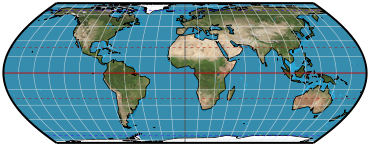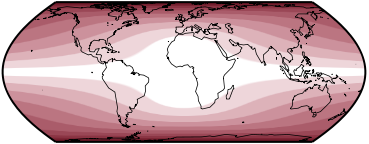## Directory of Map Projections

What is a projection?

Previous | Next

simple equal-area homotopyParameters: Initial projection, Weight of terminal projection, Standard parallel

Classifications

pseudocylindric
equal-area

Graticule

Meridians: Portions of sinusoids except when initial projection is cylindric equal-area and weight of terminal projection is 0, or initial projection is sinusoidal and weight of terminal projection is 1. In those cases, the projection is cylindric projection and so has straight meridians.
Parallels: Straight parallel lines.
Poles: Points if initial projection is cylindric equal-area and weight of terminal projection is 1, or initial projection is sinusoidal and weight of terminal projection is not 1. Lines otherwise.
Symmetry: About the central meridian and the equator.

Scale

Scale will be correct on the central meridian at two points equidistant from the equator. Depending on parameterization, the equator or two opposite parallels can have true scale.

Distortion

Depends on parameterization.

Parameters

Initial projection: Pick one of cylindric or sinusoidal projection. Each choice gives a distinct continuum between the two projections.
Weight of terminal projection: How much the projection will be skewed to projection not chosen as the initial projection.
Standard parallel: The standard parallel of the cylindric equal-area projection that will be used. This parallel will not have true scale throughout most of the continuum.

Similar projections

The Urmayev I also creates a continuum of equal-area projections between cylindric and sinusoidal.

Origin

Daniel “daan” Strebe, 2017.

Description ©2010–2020 Mapthematics LLC.